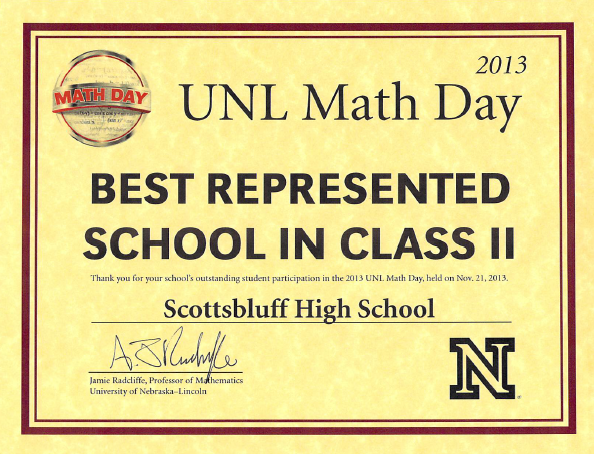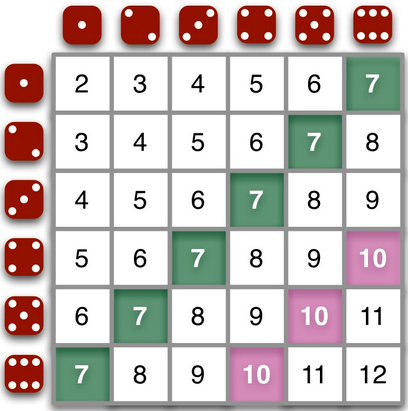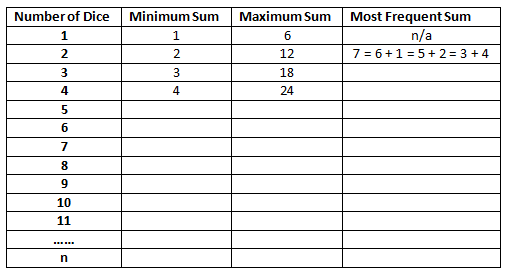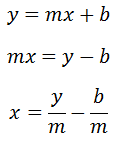# Awesome Bowl Question: Segments in a Cube

We held our first bowl team session in MTPS today: an friendly, informal competition dividing the class into two groups of roughly equal 'ability.' The bowl competition we attend at the University of Nebraska-Lincoln in November consists of games of 15 questions with 30 seconds to answer each question.

Here is a question we spent some time on in class today.Here is a screenshot from my Promethean board of the work we did as a group with students:And here is a screenshot from the Geogebra sheet students constructed in front of the class at my computer terminal to demonstrate what is going on in this problem. If you would prefer to download the Geogebra sheet for your own tinkering, you can find it here.No one got this question right in the allotted 30 seconds, so we spent some time developing the various parts of the expression. We color coded the edges on the cube (segments AB, AD, and AG) orange. We color coded the diagonals on faces of the cube (segments AC, AH, and AJ) green. We color coded the segment inside the cube (segment AI) black.

The kids had a lot of fun with building the Geogebra sheet and then trying to reconcile between sqrt(2) and the crudely rounded segment length 1.41. Or the sqrt(3) and 1.732.

The pink stuff at the top of Promethean board screen shot is our efforts to generalize this question. I tell the kids we are trying to "hack all possible problems." We use the term invariant and that we are looking to write a question that covers all possible question types. For example, what would happen to the question if s = 2? Or if s=3? Or if s=n? Is it possible to answer the question before the moderator finishes reading it? If so, at what point can we be confident we can buzz in and answer correctly?

# Nested Cylinders and Water Problem (UNL Math Day 2013 Probe I)

Here is a problem I have been working on this afternoon. I worked on building a model in Geogebra to help students understand the situation.

2013 Probe I Problem #3
A cylindrical can with radius of 6 inches is filled with 2 inches of water. When a smaller cylindrical can 4 inches tall is placed inside of the first with its bottom lying on the bottom of the first, the water in the first can rises a further inch to 3 inches. What is the radius of the smaller can?

A. 3   B. sqrt(12)   C. sqrt(15)   D. 4   E. sqrt(18)

Here is a screenshot of the Geogebra model I constructed.Here's a PDF of my typed-up solution.

Nested Cylinders Problem

Here's the Geogebra Sheet (built in the Geogebra 5.0 beta with 3-D graphing) if you'd like to take a look.

# UNL Math Day Highlights 2013

Below are some of the highlights from our UNL Math Day trip last week.Our group boards the bus in Scottsbluff. At the opening ceremony, Scottsbluff High School was recognized for driving the longest distance to the contest (400 miles!) and for being the best represented school in Class II with 50 students.Our certificate for being the best represented school.Another group of 24 students sat in on a math capstone course for undergraduates that intend to become secondary math educators. UNL Professor Yvonne Lai invited SHS students to speak about the special mathematics curriculum students enjoy in the SHS Math Theory and Problem Solving class. A group of teaching methods students (center semicircle) talk to SHS students about college opportunities and the value of mathematics.Andrew and Kyle address the capstone class. Pictured is the UNL Math Day promotional poster that Andrew (left) designed for the University.Our students (left) compete in the Math Bowl competition. We ended up losing this match to Lincoln Pius X - had a chance to tie it on the last question! - 7 to 6. In the second round, we lost on a tiebreaker. Despite the setbacks, the students really had fun preparing for and participating in the competition.

# (Not So Typical) Dice Problems • 1 Comment

Probability and counting questions associated with rolling two dice are frequent at math contests. For example, one might ask, "What is the most common sum when two dice are rolled?" The grid below helps the student see the most common sum.Exhibit A: The sums of two dice. (Ignore the pink stuff; 7 is the most common sum).

Suppose we construct the probability distribution for rolling two dice.Exhibit B: Probability simulation for rolling two dice. 7 is the most likely sum.

One of my colleagues has 11 dice in her classroom. This made me wonder what would be the most likely sum for 11 dice. How would we go about answering this question?

Problems like this one show students the value of breaking a larger problem into smaller problems. Let's construct a table for the minimum and maximum sums for rolling n number of dice and see if we can determine some patterns. Our aim is to generalize our findings to n dice.Exhibit C: A preliminary table for rolling n dice.

How would you support students in working towards the generalization? For example, modeling two dice with a table is fairly simple, as shown above. What about modeling three dice? Sure, you could go three dimensional... but what about four dice? Five dice? This problem poses some great modeling questions.Exhibit D: Polynomial approach to obtaining dice counts for the case where n = 2.

# Geometry Week Day 4: y = mx + b, the x-Intercept, and Bowl Contest Problems • 1 Comment

Gesturing is a powerful way for students to learn. I have students gesture with their hands often, particularly when we examine behavior of graphs. One of our teachers was working to help students remember the slope-intercept form of a line. She found this video on Youtube:

The teacher had the students do the gestures to help remember the parts of the slope-intercept form. I wanted to help the students understand the graphical effects of changing the values of m and b on the line y = mx + b. I started with a blank Geogebra sheet and constructed the graph of y = mx + b actively in front of the students.Students had no trouble with the effect altering the value of m had on the graph. However, something interesting happened when I asked the class, "What is your prediction for the effect changing b will have on the graph?" One of the students quickly replied, "The black point will move along the x-axis." I think my face betrayed my attempt to remain neutral. I said with my mouth, "You're right, the black point will move along the x-axis. What else will happen?" The students eventually pointed out the relationship between the b-value on the slider and the y-coordinate of the pink point.

But that student's comment has stuck with me since yesterday. I hadn't really considered before how we would go about defining the movement of the black point along the x-axis.Let's take a second look at the graph.